SPSS TUTORIALS BASICS ANOVA REGRESSION FACTOR CORRELATION

# How to Compute Means in SPSS?

This tutorial shows how to compute means over both variables and cases in a simple but solid way. We encourage you follow along by downloading and opening restaurant.sav, part of which is shown below.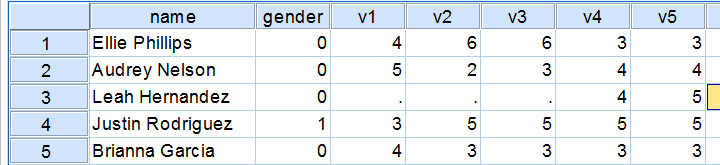## Quick Data Check

Before computing anything whatsoever, we always need to know what's in our data in the first place. Skipping this step often results in ending up with wrong results as we'll see in a minute. Let's first inspect some frequencies by running the syntax below.

*Show data values and value labels in output tables.
set tnumbers both.

*Quick data check.
frequencies v1 to v5.

## Result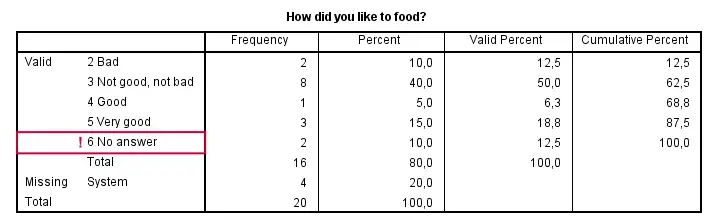Right, now there's two things we need to ensure before proceeding. Firstly, do all variables have similar coding schemes? For the food rating, higher numbers (4 or 5) reflect more positive attitudes (“Good” and “Very good”) but does this hold for all variables? If we take a quick peek at our 5 tables, we see this holds.
Second, do we have any user missing values? That is, do we want to include all data values in our computations? In this case, we don't. We need to exclude 6 (“No answer”) from all computations. We'll do so with the syntax below.

## Setting Missing Values

*Set 6 as user missing value.
missing values v1 to v5 (6).

*Check again.
frequencies v1 to v5.

## Result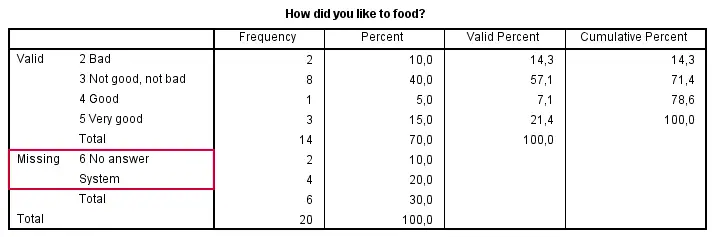## Computing Means over Variables

Right, the simplest way for computing means over variables is shown in the syntax below. Note that we can usually specify variable names separated by spaces but for some odd reason we need to use commas in this case.

*Compute mean over v1, v2, v3, v4 and v5.

compute happy1 = mean(v1, v2, v3, v4, v5).
execute.

If our target variables are adjacent in our data, we don't need to spell out all variable names. Instead, we'll enter only the first and last variable names (which can be copy-pasted from variable view into our syntax window) separated by TO.

*Alternative: use TO keyword for specifying variables.

compute happy2 = mean(v1 to v5).
execute.

## Computing Means - Dealing with Missing Values

If we take a good look at our data, we see that some respondents have a lot of missing values on v1 to v5. By default, the mean over v1 to v5 is computed for any case who has at least one none missing value on those variables. If all five values are (system or user) missing, a mean can't be computed so it will be a system missing value as we see in our data.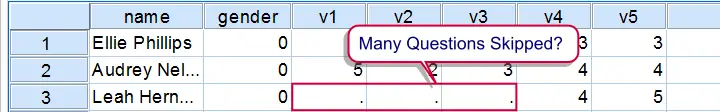It's quite common to exclude cases with many missings from computations. In this case, the easiest option is using the dot operator. For example `mean.3(v1 to v5)` means “compute means over v1 to v5 but only for cases having at least 3 non missing values on those variables”. Let's try it.

## Computing Means - Exclude Cases with Many Missings

*Compute mean only for cases having at least 3 valid values over v1 to v5.

compute happy3 = mean.3(v1 to v5).
execute.

## Result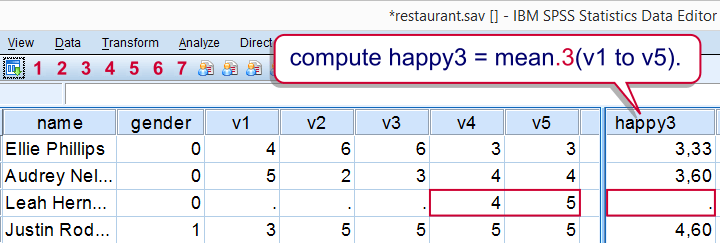A more general way that'll work for more complex computations as well is by using IF as shown below.

*Alternative way to exclude cases having fewer than 3 valid values over v1 to v5.

if (nvalid (v1 to v5) >= 3) happy4 = mean(v1 to v5).
execute.

## SPSS - Compute Means over Cases

So far we computed horizontal means: means over variables for each case separately. Let's now compute vertical means: means over cases for each variable separately. We'll first create output tables with means and we'll then add such means to our data.
Means over all cases are easily obtained with DESCRIPTIVES as in descriptives v1 v2.

## Result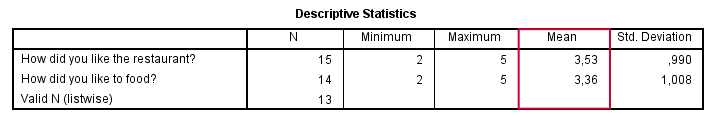## Means for Groups Separately

So what if we want means for male and female respondents separately? One option is SPLIT FILE but this is way more work than necessary. A simple MEANS command will do as shown below.

*Show only value labels (no data values) in output tables.

set tnumbers labels.

*Report means for genders separately.

means v1 v2 by gender/cells means.

## Result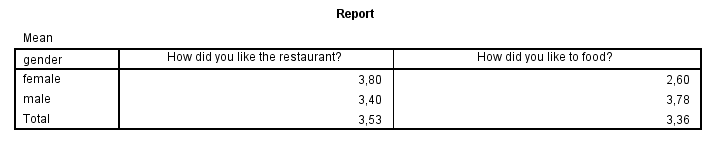## SPSS - Add Means to Dataset

Finally, you may sometimes want means over cases as new variables in your data. The way to go here is AGGREGATE as shown below.

*Add mean over v1 as new variable to data.

/mean_1 = mean(v1).

If you'd like means for groups of cases separately, add one or more `BREAK` variables as shown below. This example also shows how to add means for multiple variables in one go, again by using TO.

*Add means over v2 to v5 for genders separately as new variables to data.

/break gender
/mean_2 to mean_5 = mean(v2 to v5).

## Result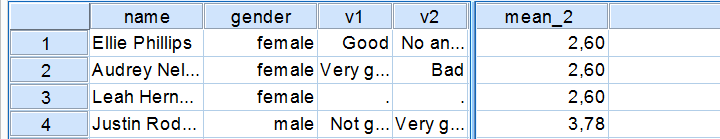Note that we already saw these means (over v2, for genders separately) in our output after running means v2 by gender.
Right. That's about all we could think of regarding means in SPSS. If you've any questions or remarks, please feel free to throw in a comment below.

# Tell us what you think!

*Required field. Your comment will show up after approval from a moderator.

# THIS TUTORIAL HAS 11 COMMENTS:

•### By JL Euste on April 2nd, 2017

How can I add means FOR GROUPS to the data set? You only included calculating means for groups reported as a table, but I want the means for groups as a new variable. Thank you!

•### By Ruben Geert van den Berg on April 2nd, 2017

Hi Euste! Use AGGREGATE with one or more BREAK variables. For example

/BREAK GENDER
/mean_salary = mean(salary).

will add mean salaries for genders separately to your dataset. They'll be the same means as you'll get from

means salary by gender.

•### By Loo on October 27th, 2018

Hi Ruben,
Ten subjects have been tested by the same measure (imagine an intelligence test). But, there is different number of taking part in experiment for each subject (e.g., Subject A: 1 times; Subject B: 7 times; Subject C: 2 times; etc.). Is the mean of means the best option for calculating the mean scores? If yes then how I should calculate its SD?

•### By Ruben Geert van den Berg on October 28th, 2018

Hi Loo!

I'd compute both the mean and standard deviation over the tests they've taken. Assuming the tests are variables (not cases) in your data, use something like

`compute m1 = mean(q1 to q5).`

and

`compute s1 = sd(q1 to q5).`

and then give them clear variable labels: `variable labels m1 'Mean score over tests taken'.`

Both functions use the sums over valid values and divide by the numbers of valid values -which is the right way to go for the vast majority of cases.

Hope that helps!

•### By Loo on October 29th, 2018

Thank you so much for reply. Actually I want to know your idea. Here is my data:
Subject - score
A: 6, 6
B: 7
C: 7
D: 6
E: 6, 5, 6, 6, 7, 6, 6, 7, 6, 7
F: 5, 5, 5
I need the mean for all of these scores, and I want to use this formula (the mean of means) for it:
M = M1 + M2 + M3 + M4 + M5 + M6 / 6
Do you think using the mean of means is correct here? Then could you please simply write its SD formula?